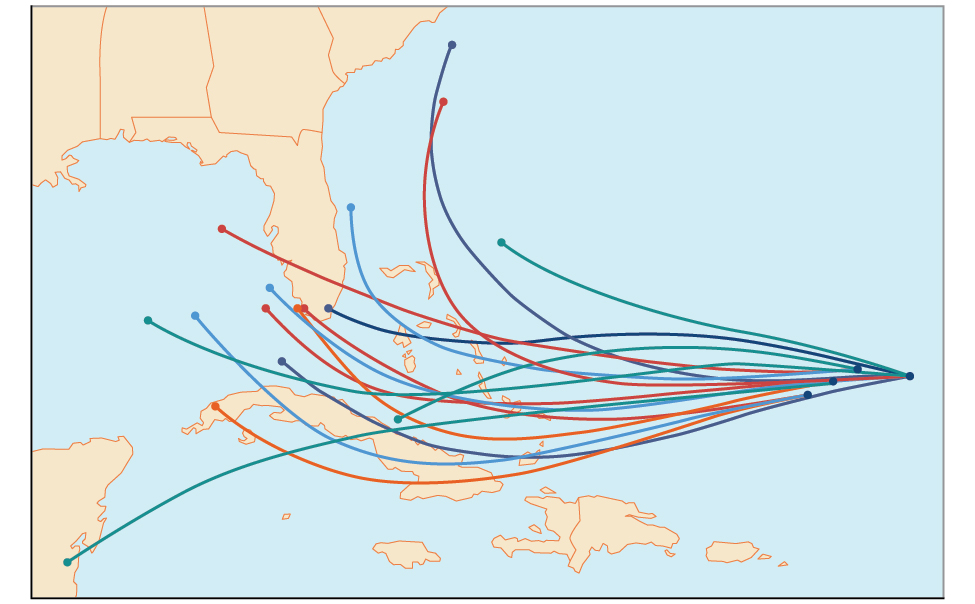# 11.7 Probability

 Page 1 / 18
In this section, you will:
• Construct probability models.
• Compute probabilities of equally likely outcomes.
• Compute probabilities of the union of two events.
• Use the complement rule to find probabilities.
• Compute probability using counting theory.An example of a “spaghetti model,” which can be used to predict possible paths of a tropical storm. The figure is for illustrative purposes only and does not model any particular storm.

Residents of the Southeastern United States are all too familiar with charts, known as spaghetti models, such as the one in [link] . They combine a collection of weather data to predict the most likely path of a hurricane. Each colored line represents one possible path. The group of squiggly lines can begin to resemble strands of spaghetti, hence the name. In this section, we will investigate methods for making these types of predictions.

## Constructing probability models

Suppose we roll a six-sided number cube. Rolling a number cube is an example of an experiment    , or an activity with an observable result. The numbers on the cube are possible results, or outcomes    , of this experiment. The set of all possible outcomes of an experiment is called the sample space    of the experiment. The sample space for this experiment is $\left\{1,2,3,4,5,6\right\}.\text{\hspace{0.17em}}$ An event    is any subset of a sample space.

The likelihood of an event is known as probability    . The probability of an event $p$ is a number that always satisfies $0\le p\le 1,$ where 0 indicates an impossible event and 1 indicates a certain event. A probability model    is a mathematical description of an experiment listing all possible outcomes and their associated probabilities. For instance, if there is a 1% chance of winning a raffle and a 99% chance of losing the raffle, a probability model would look much like [link] .

Outcome Probability
Winning the raffle 1%
Losing the raffle 99%

The sum of the probabilities listed in a probability model must equal 1, or 100%.

Given a probability event where each event is equally likely, construct a probability model.

1. Identify every outcome.
2. Determine the total number of possible outcomes.
3. Compare each outcome to the total number of possible outcomes.

## Constructing a probability model

Construct a probability model for rolling a single, fair die, with the event being the number shown on the die.

Begin by making a list of all possible outcomes for the experiment. The possible outcomes are the numbers that can be rolled: 1, 2, 3, 4, 5, and 6. There are six possible outcomes that make up the sample space.

Assign probabilities to each outcome in the sample space by determining a ratio of the outcome to the number of possible outcomes. There is one of each of the six numbers on the cube, and there is no reason to think that any particular face is more likely to show up than any other one, so the probability of rolling any number is $\text{\hspace{0.17em}}\frac{1}{6}.$

 Outcome Roll of 1 Roll of 2 Roll of 3 Roll of 4 Roll of 5 Roll of 6 Probability $\frac{1}{6}$ $\frac{1}{6}$ $\frac{1}{6}$ $\frac{1}{6}$ $\frac{1}{6}$ $\frac{1}{6}$

Do probabilities always have to be expressed as fractions?

No. Probabilities can be expressed as fractions, decimals, or percents. Probability must always be a number between 0 and 1, inclusive of 0 and 1.

what is set?
a colony of bacteria is growing exponentially doubling in size every 100 minutes. how much minutes will it take for the colony of bacteria to triple in size
I got 300 minutes. is it right?
Patience
no. should be about 150 minutes.
Jason
It should be 158.5 minutes.
Mr
ok, thanks
Patience
100•3=300 300=50•2^x 6=2^x x=log_2(6) =2.5849625 so, 300=50•2^2.5849625 and, so, the # of bacteria will double every (100•2.5849625) = 258.49625 minutes
Thomas
what is the importance knowing the graph of circular functions?
can get some help basic precalculus
What do you need help with?
Andrew
how to convert general to standard form with not perfect trinomial
can get some help inverse function
ismail
Rectangle coordinate
how to find for x
it depends on the equation
Robert
yeah, it does. why do we attempt to gain all of them one side or the other?
Melissa
whats a domain
The domain of a function is the set of all input on which the function is defined. For example all real numbers are the Domain of any Polynomial function.
Spiro
Spiro; thanks for putting it out there like that, 😁
Melissa
foci (–7,–17) and (–7,17), the absolute value of the differenceof the distances of any point from the foci is 24.
difference between calculus and pre calculus?
give me an example of a problem so that I can practice answering
x³+y³+z³=42
Robert
dont forget the cube in each variable ;)
Robert
of she solves that, well ... then she has a lot of computational force under her command ....
Walter
what is a function?
I want to learn about the law of exponent
explain this
what is functions?
A mathematical relation such that every input has only one out.
Spiro
yes..it is a relationo of orders pairs of sets one or more input that leads to a exactly one output.
Mubita
Is a rule that assigns to each element X in a set A exactly one element, called F(x), in a set B.
RichieRich

#### Get Jobilize Job Search Mobile App in your pocket Now!ByBy Brooke DelaneyBy OpenStaxBy Kimberly NicholsBy OpenStaxBy Rohini AjayBy JavaChamp TeamBy Brooke DelaneyBy Janet ForresterBy Ann SchlosserBy OpenStax